Quantum Objects

In the last quiz, we encountered head-on the measurement problem in quantum mechanics: we could not devise an experiment capable of measuring the neutron spin components without destroying previously collected information along the way. It appears that the experimental apparatus we’re using must disturb the neutron spins while observing them, making it impossible to precisely determine the direction of the spin vector. This interesting consequence of quantum objects is not limited to spin, as we’ll see in later chapters.

How might we be disturbing the neutrons? Since this quantum measurement problem is generally only observed with microscopic systems, we might guess that our macroscopic magnets generating the magnetic field $\mathbf{B}$ are simply too strong: it's easy to imagine measuring the speed of a baseball with a radar gun, but difficult to imagine measuring the speed of a neutron, whether or not quantum mechanics governs its motion.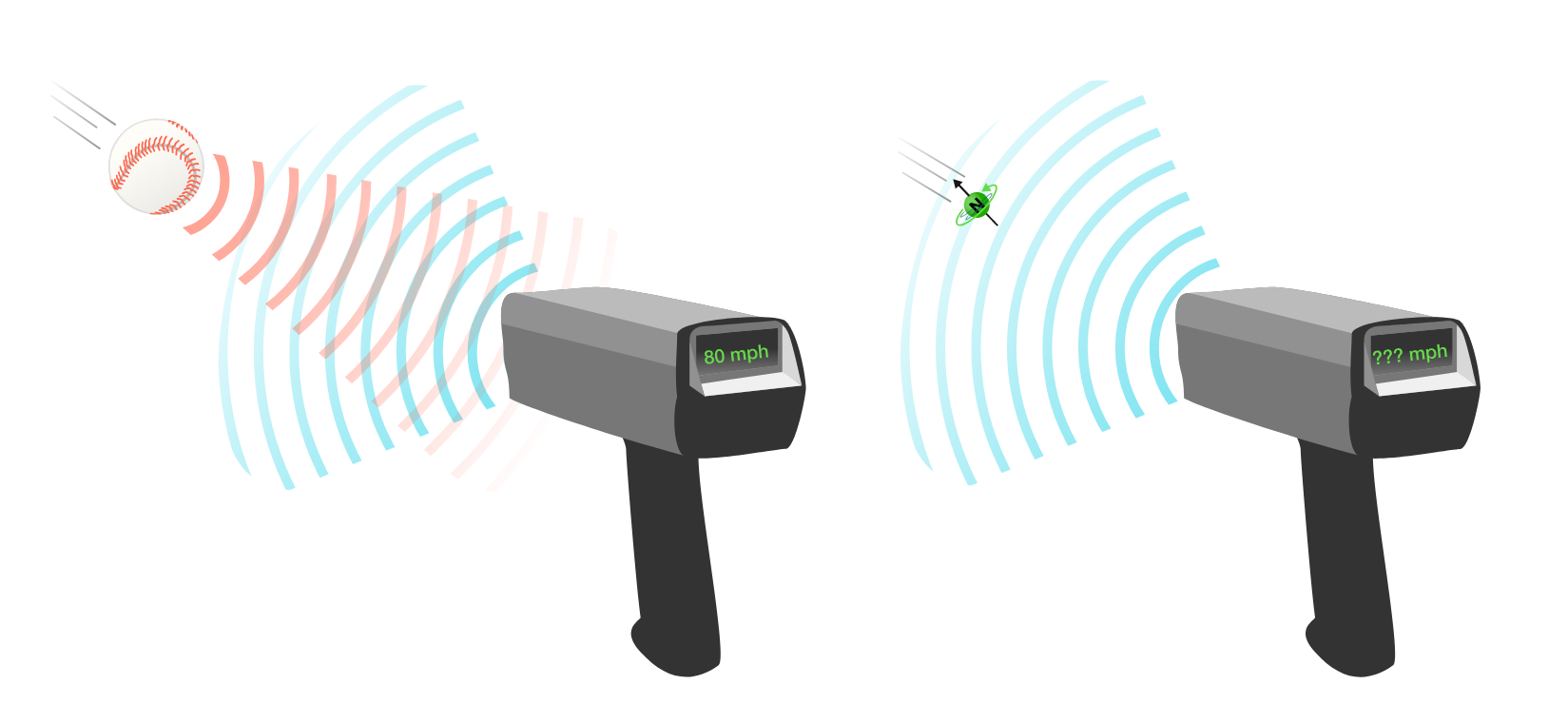Let’s investigate this measurement problem a bit more. Can we determine what step in the SG experiment is disturbing the state of our neutrons?

Measurement and Memory

Let’s revisit the experimental setup with three SG analyzers. The first SG-$z$ analyzer filters the unknown state $\ket{\psi}$ into two possibilities: $\ket{\uparrow}$ and $\ket{\downarrow}$, corresponding to “up” and “down” spin neutrons. We’ve now set up our apparatus so that 100 $\ket{\uparrow}$ neutrons are prepared by the first analyzer.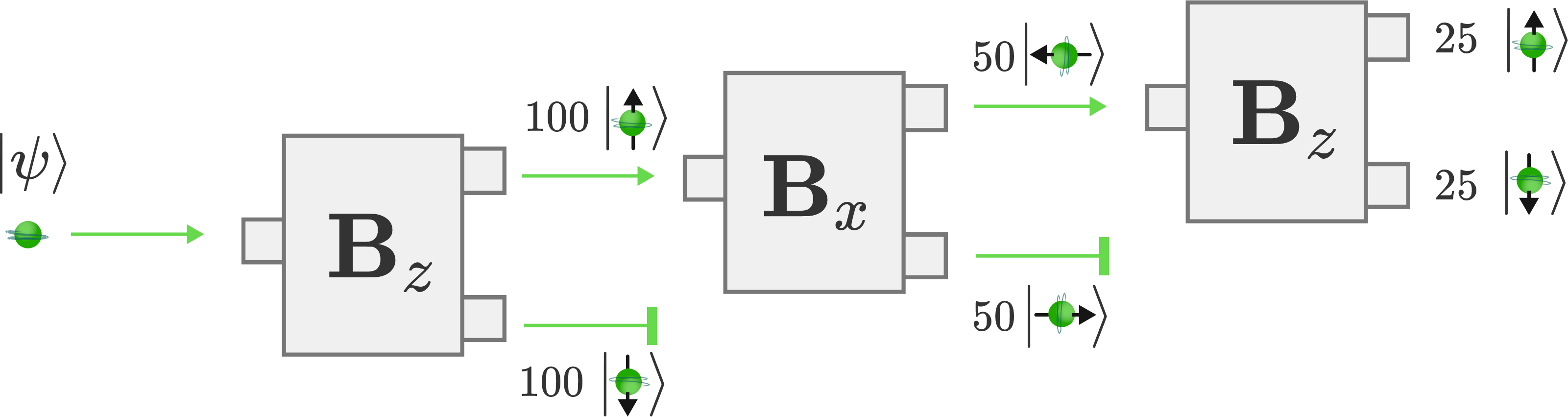The output from this first analyzer is directed towards the input of an SG-$x$ analyzer. Measuring the $x$-component of spin is incompatible with the $z$ measurement we’ve already performed, so this analyzer resets the neutrons into two states: $\ket{\rightarrow}$ and $\ket{\leftarrow}$, aligned parallel and anti-parallel with the $x$-axis.

The state that an incident $\ket{\uparrow}$ neutron collapses into is probabilistic, and so with 100 neutrons input into the analyzer, we would expect 50 neutrons in the $\ket{\rightarrow}$ channel, and 50 with the $\ket{\leftarrow}$ channel.

Finally, we direct the $\ket{\leftarrow}$ channel into a third SG-$z$ analyzer. Even though these neutrons have been measured previously as $\ket{\uparrow}$, that knowledge has been permanently erased by measuring an incompatible observable. Instead of confirming the $\ket{\uparrow}$ spin of all $50$ or these neutrons, we again observe a reset: 25 neutrons in the $\ket{\uparrow}$ channel, and 25 in the $\ket{\downarrow}$ channel.

Measurement and Memory

We’ve made a slight variation to our experimental apparatus.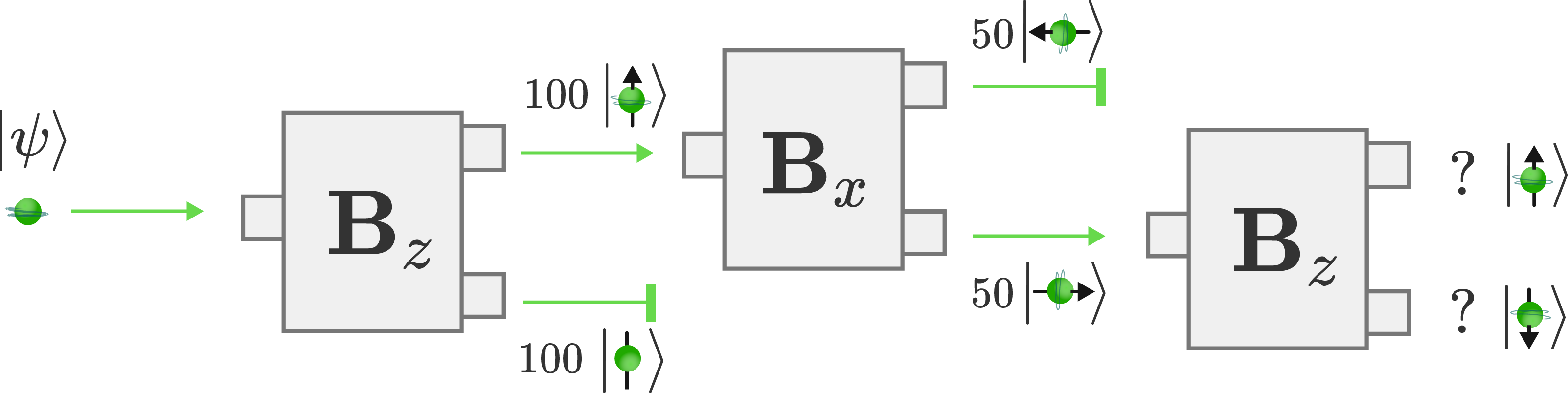Instead of measuring the $\ket{\leftarrow}$ channel, we've selected the $\ket{\rightarrow}$ channel and used that as input for the third analyzer.

Given what we've learned about incompatible measurements destroying previously collected information, what do we expect to output from the third SG device?

Measurement and Memory

Measuring the $x$-component of the spin destroys any previously collected knowledge of the spin state. So whether or not you analyze the $\ket{\rightarrow}$ or $\ket{\leftarrow}$ channels, you still have no information about the $z$-component, so you get a random $50:50$ distribution.

Let’s now combine the $\ket{\rightarrow}$ and $\ket{\leftarrow}$ beams of neutrons which emerge from the second SG analyzer. The exact experimental method we use to perform this combination isn’t important; we use an arbitrary set of magnets to deflect the beams back into alignment.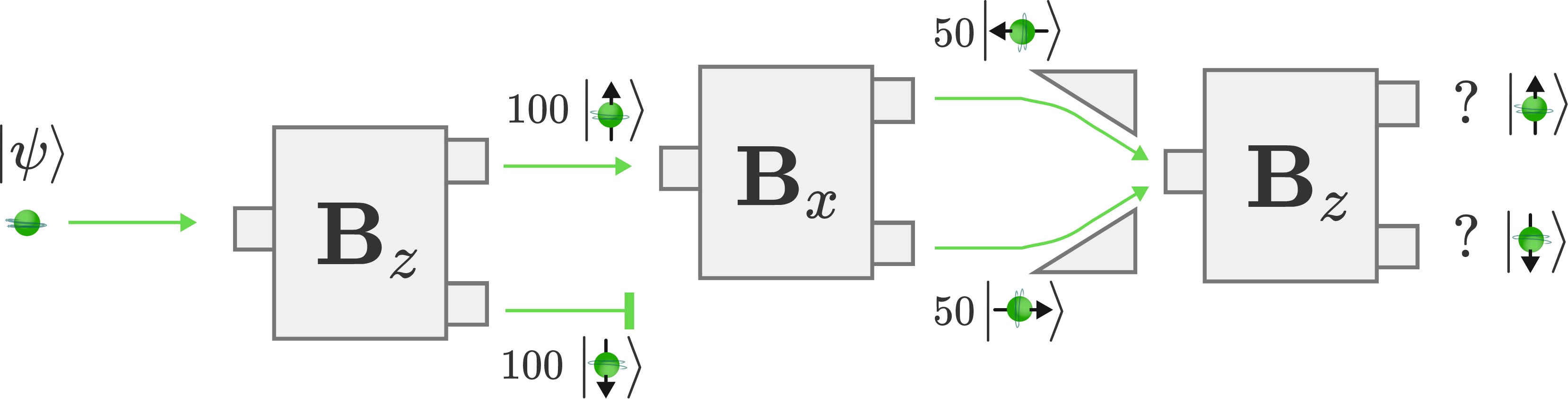Measurement and Memory

Can we tease out what the composition of this mixed state is based on the two experiments we just looked at?

We can assume that since it went through the $x$-aligned SG analyzer, we’ve already disturbed the system and presumably lost any information about the $z$-alignment of the neutrons. The neutrons leaving the $x$-aligned analyzer have been reset into $\ket{\rightarrow}$ and $\ket{\leftarrow}$ states. We must assume that the set of magnets used to realign the two deflected beams did not further disturb the alignment of the neutrons.Based on the two previous experiments, what do you expect the result of the third SG analyzer to be?

Measurement and Memory

This is not what we observe. The result is classically absurd, and contradicts our expectations: all of the neutrons emerge from the $\ket{\uparrow}$ exit of the third analyzer.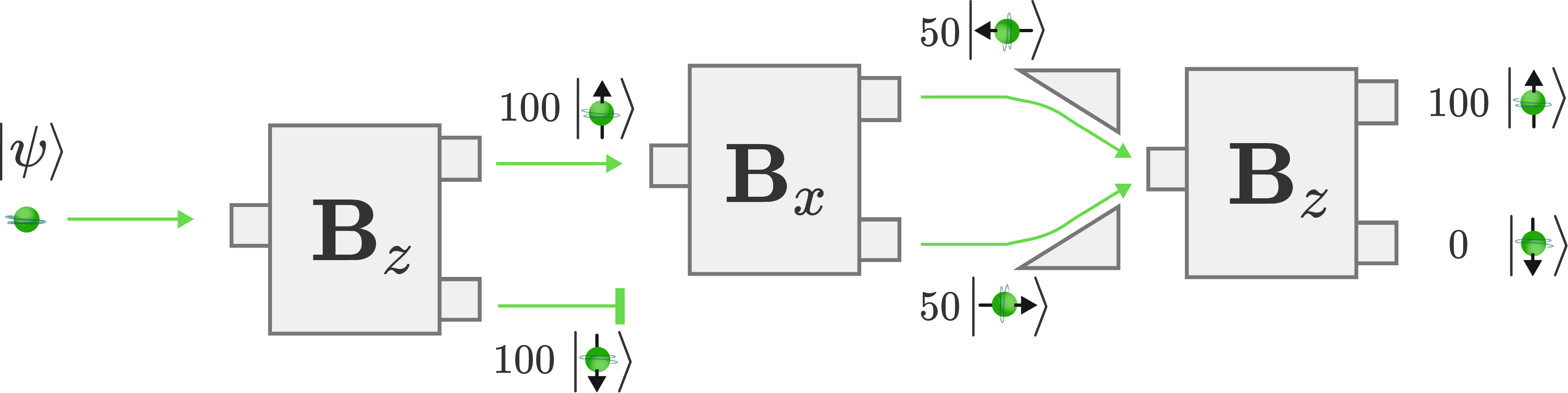What could explain this observation?

Measurement and Memory

The measurement problem is more sinister than a practical disturbance caused by incident magnetic fields. What we thought might be a shortcoming of our experimental device turned out to be the reflection of something much deeper: it's the act of measurement itself that is changing the state of our neutrons.Though the neutrons went through the SG-$x$ analyzer in the experiment above, their $x$-components were never actually observed, and were recombined so that the channels could not be distinguished again. From the point of view of the neutrons, they were never observed, and no incompatible measurements have been performed that would reset their states. The role of observers in quantum mechanical measurement is completely unique and has no classical equivalent. This fundamental quantum mechanical property is the most important we've learned so far:

Observers can change the state of quantum objects. Quantum states are reset by observation itself, though the point in the experiment when this occurs is still the subject of active debate.

Measurement and Memory

This counterintuitive result of combining spin channels also reveals an important caveat to keep in mind when analyzing quantum systems:

The probabilistic behavior of quantum objects is not limited to SG analyzers, and it's often tempting to break a quantum system down into simpler independent paths and combine their probabilities.

We can try to understand the combined channel experiment by analyzing two single channel experiments, and note the probability of a neutron emerging in each state: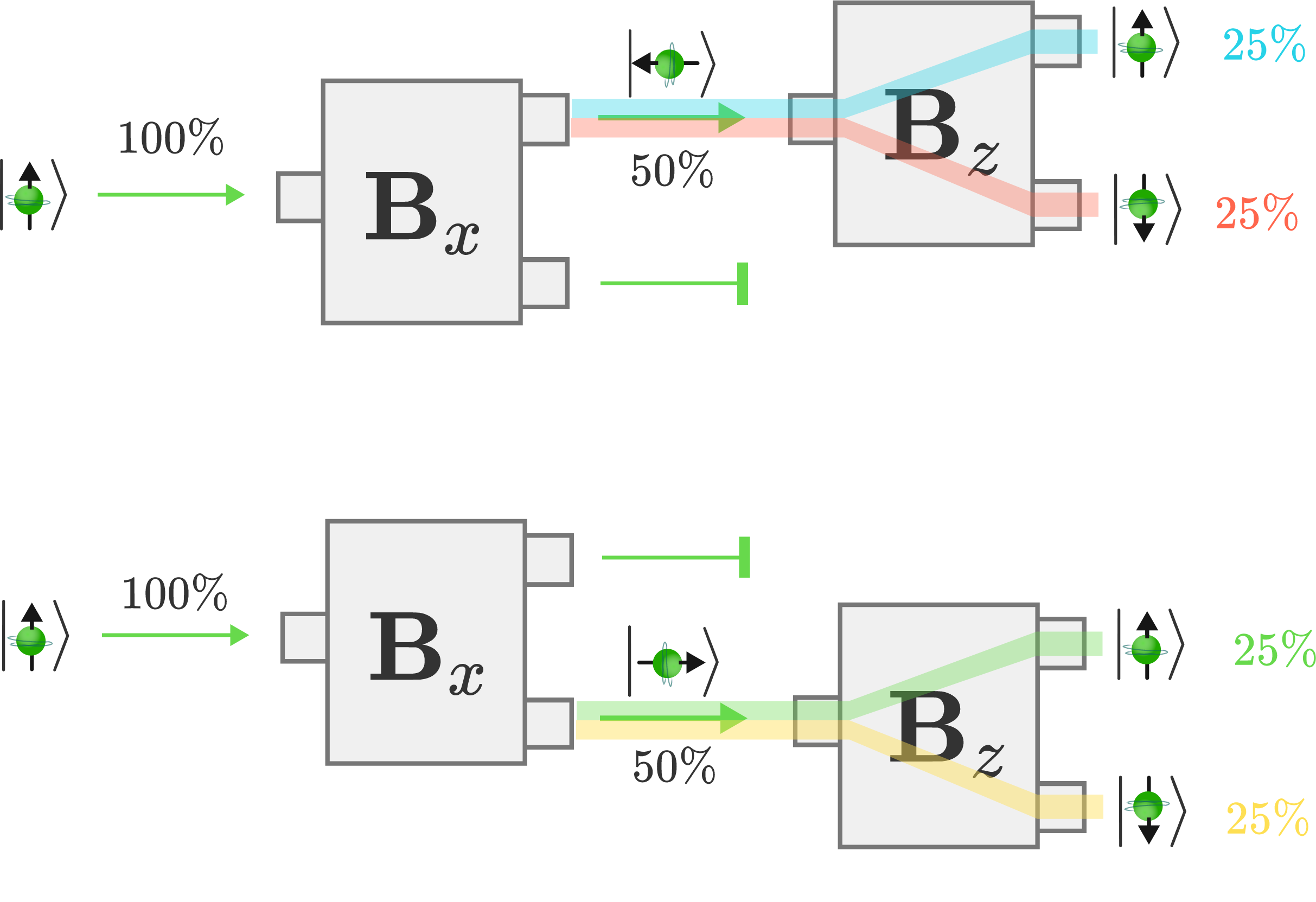But when we put these two results together and see what they predict for each channel of the combined experiment, we reach a glaring contradiction: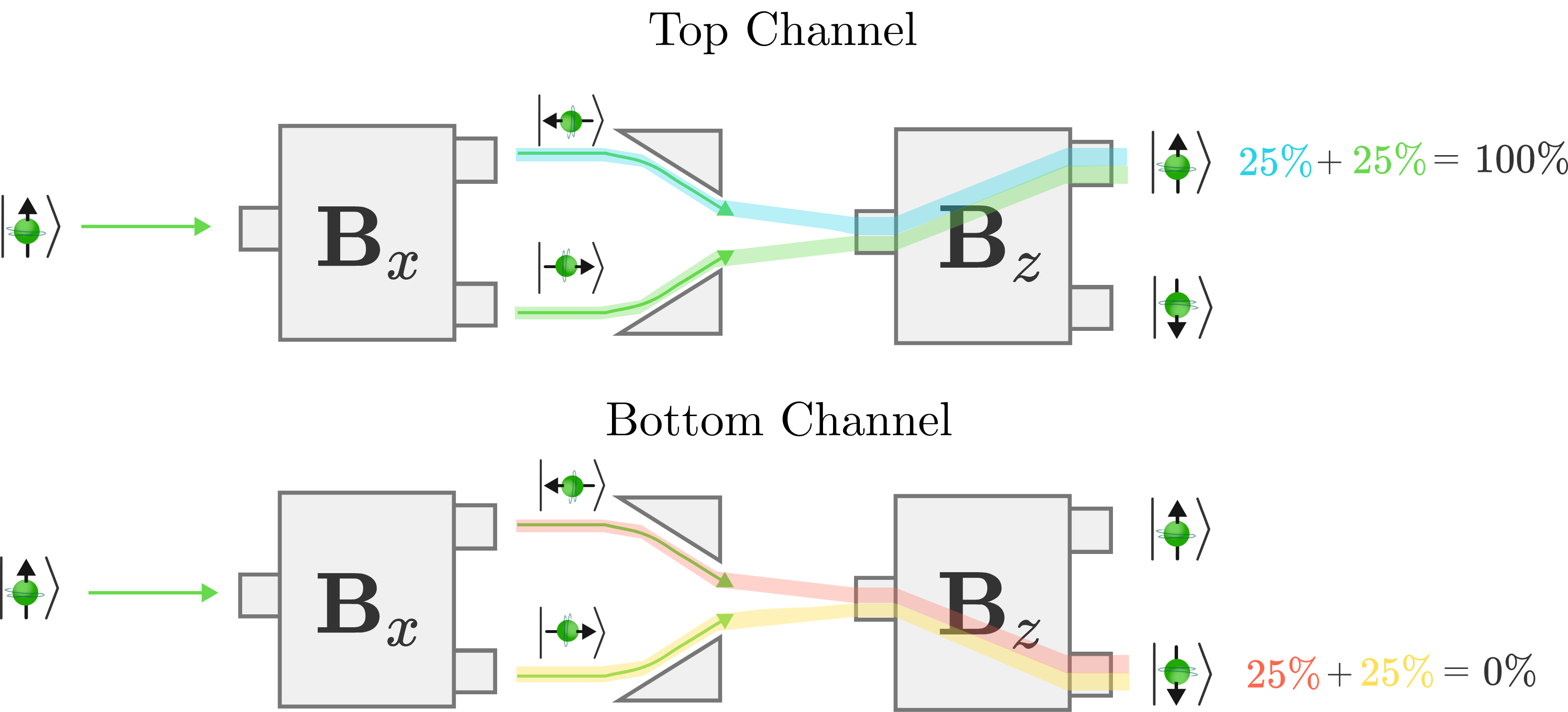What should we conclude from these results?

Measurement and MemoryIn the single channel experiments, $50\%$ of the neutrons are blocked after the second analyzer and $25\%$ of the neutrons exit the $\ket{\downarrow}$ channel of the third analyzer. In the combined channel experiment, 100% of the neutrons pass from the second analyzer to the third analyzer, yet fewer neutrons come out in the $\ket{\downarrow}$ channel. In fact, there are no neutrons in that channel at all!

This combined experiment allowed the neutrons more paths to reach the final $\ket{\downarrow}$ channel but ends up with fewer neutrons making it. It's as though we opened a second window to a room and part of the room got darker: classical probability can't hope to explain this part of quantum mechanics known as interference, in which combining two effects can lead to cancellation, rather than enhancement. Interference will play an essential role in our later adventures with quantum objects.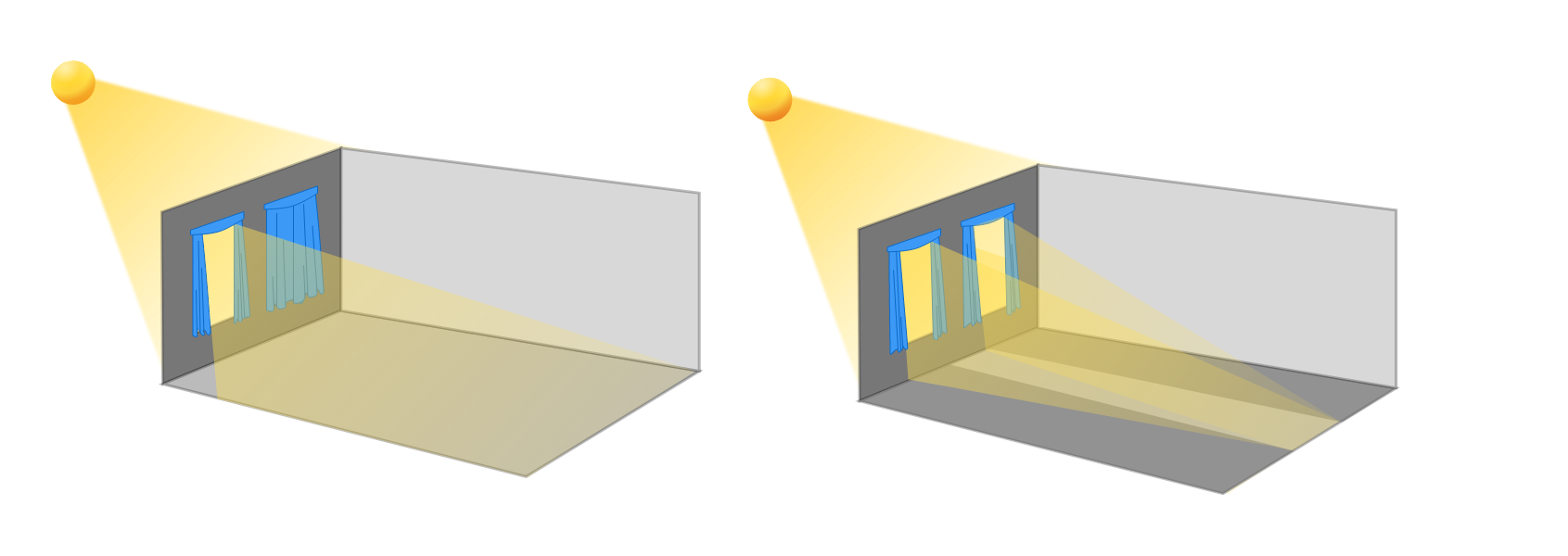Measurement and Memory

×